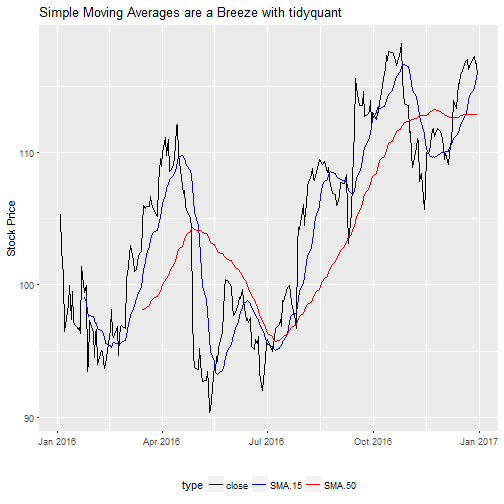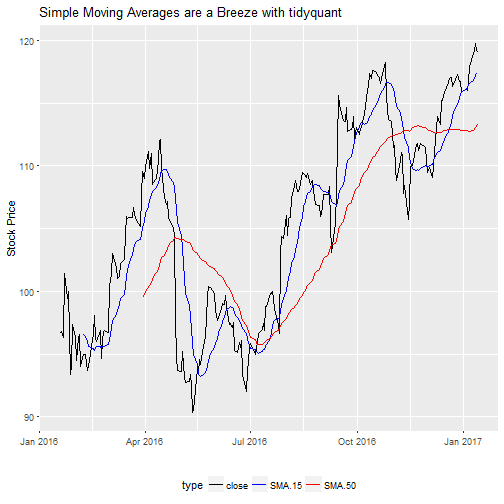# tidyquant: Bringing Quantitative Financial Analysis to the tidyverse

WrittenMy new package, tidyquant, is now available on CRAN. tidyquant integrates the best quantitative resources for collecting and analyzing quantitative data, xts, quantmod and TTR, with the tidy data infrastructure of the tidyverse allowing for seamless interaction between each. While this post aims to introduce tidyquant to the R community, it just scratches the surface of the features and benefits. We’ll go through a simple stock visualization using ggplot2, which which shows off the integration. The package is open source, and you can view the code on the tidyquant github page.

• 2017-01-17: I updated the post to use the 0.2.0 tq_mutate and tq_transform function arguments ohlc_fun, x, and y (replaces x_fun, .x and .y, respectively). These will be deprecated in 0.3.0 so please make the switch! :)

# Why tidyquant?

One of the reasons why I began my journey into R programming is because it’s the best open-source option for stock analysis. With quantitative financial analysis (QFA) packages like quantmod, xts and TTR, stock data can quickly be retrieved, sliced and diced, transformed and mutated, and visualized so I can make investment decisions. It’s really a beautiful thing.

Over time, the R programming landscape has evolved. A major step forward was the tidyverse, a collection of R packages that work in harmony, are built for scale-ability, and are well documented in R for Data Science. However, a problem has surfaced: the QFA packages are not easy to use with the tidyverse. The tidyverse works with data frames while the QFA packages work with extensible time-series (xts) objects. Both are great, but they don’t easily work together.

As you can imagine, my workflow was longer than I’d like. I’d work in xts to use various functions to calculate moving averages, moving average convergence divergence (MACD), Bollinger Bands, etc, and then convert to tibbles (tidy dataframes) for mapping functions with purrr to scale to many stocks, for mutating dataframes with dplyr to add new columns, and for visualizing my analysis using ggplot2. This got very long and repetitive…

Enter tidyquant. The package started off as a collection of scripts aimed at increasing my efficiency and performance of my stock analyses:

• I would start by getting data with tq_get(), which returns data, such as stock prices or financial statements, as a tibble object.
• I’d use tq_transform() to use the various quantmod and xts functions that can change periodicities, such as period returns and conversion from daily to monthly periodicity.
• I’d use tq_mutate() to seamlessly apply the various TTR functions, such as moving averages, MACD’s, Bollinger Bands, etc.
• And, I’d do all of this without ever leaving the tidyverse, which allowed me to mutate, pipe (%>%), and scale my analyses at ease.

In this evolution and in the spirit of open source, I have released the tidyquant package to the R community with the hope that others can benefit from the integration between the QFA packages (quantmod, xts, and TTR) and the tidyverse. I believe this is the right way to go, and I’m looking forward to hearing your feedback.

# Benefits

The tidyquant philosophy:

• A few core functions with a lot of power, that
• leverage the quantitative analysis power of xts, quantmod and TTR, and are
• designed to be used and scaled with the tidyverse.

# Example: Visualizing Moving Averages

I’ll go through an example of visualizing the 15-day and 50-day moving averages of the stock symbol, AAPL, which is for Apple Inc. Moving averages are a popular trading tool that stock analysts use to determine buying and selling signals. According to Investopedia, the moving average is…

A widely used indicator in technical analysis that helps smooth out price action by filtering out the “noise” from random price fluctuations. A moving average (MA) is a trend-following or lagging indicator because it is based on past prices. The two basic and commonly used MAs are the simple moving average (SMA), which is the simple average of a security over a defined number of time periods, and the exponential moving average (EMA), which gives bigger weight to more recent prices. The most common applications of MAs are to identify the trend direction and to determine support and resistance levels. While MAs are useful enough on their own, they also form the basis for other indicators such as the Moving Average Convergence Divergence (MACD).

Of particular interest is the crossover, the point at which a trend begins to emerge, which can be used as a buy or sell signal.Let’s go through an example to visualize the 15-day and 50-day moving averages for AAPL.

## Step 1: Prerequisites

The tidyquant package can be downloaded from CRAN:

For those following along in R, you’ll need to load the following package:

I also recommend the open-source RStudio IDE, which makes R Programming easy and efficient.

## Step 2: Use tq_get to get stock prices

We’ll start by getting the last year of stock prices. We use the tidyquant tq_get() function for all data retrieval. Set the parameter get = "stock.prices" to tell tidyquant we want the historical stock prices. We can use the from argument to pass a date as the start of the collection, which accepts character string in the form of “YYYY-MM-DD”. We can use lubridate functions today() and years() to get the date from one year ago.

We now have 251 days of stock prices as a tibble object. This is exactly the format we want for working in the tidyverse.

## Step 3: Use tq_mutate to add moving averages

We need to get the 15-day and 50-day moving averages. We want to use the SMA() function from the TTR package. To use any of these functions in the tidyverse, we have a few options with pros and cons:

• dplyr::mutate(): Used to add a single column to a data set. Only able to add a single column to a tibble. For SMA(), this works because a single column is generated. For other functions such as BBands() and MACD, multiple columns are generated that fail on mutate().

• tidyquant::tq_mutate(): Used to add single or multiple columns to a data set. Uses quantmod OHLC notation (more on this in a minute). The output generated must be the number of rows as the input dataframe (otherwise the data can’t be joined). Because multiple columns can be returned, works with BBands() and MACD().

• tidyquant::tq_transform(): Used to return a new data set with output only (does not return the input dataframe). Uses OHLC notation. Most flexible option.

• tidyquant::tq_mutate_xy(): Same as tq_mutate() but works using up to two column inputs instead of OHLC notation.

• tidyquant::tq_transform_xy(): Same as tq_transform() but works using up to two column inputs instead of OHLC notation.

For this tutorial, we will use tq_mutate() to expose you to OHLC notation along with the tidyquant function workflow. We’ll also show tq_mutate_xy() so you can see the difference in arguments.

### tq_mutate() function

tq_mutate() has two primary arguments: ohlc_fun and mutate_fun:

• ohlc_fun: Takes quantmod::OHLC functions, which are Op, Cl, Hi, Lo, Vo, Ad, HLC, OHLC, and OHLCV. The OHLC notation is the basis of all quantmod, xts, and TTR functions. These functions collect a subset of the dataframe columns matching open, high, low, close, volume, and/or adjusted. Think of the OHLC notation akin to the dplyr::select() function, which selects columns. Op selects the column named “open”, and HLC selects “high”, “low” and “close” columns.

• mutate_fun: Takes any quantmod, xts, or TTR function listed in tq_mutate_fun_options() (see below for compatible functions). The mutation_fun performs the work. Any additional parameters of the passed via ... in the tq_mutate() function go to the mutation_fun.

An example with SMA() from the TTR package helps solidify how it works. Reviewing the documentation for SMA, we see that the function, SMA(x, n = 10, ...), accepts x a price or volume and n a number of periods to average over. For the 15-day simple moving average, we would pass a set of prices, either “close” or “adjusted”, and n = 15 for 15 days. In OHLC notation ohlc_fun = Cl for “close” or ohlc_fun = Ad for adjusted. The mutate_fun = SMA, and we pass n = 15 as an additional argument. Shown below, we pipe (%>%) our tibble of AAPL stock prices to tq_mutate(ohlc_fun = Cl, mutate_fun = SMA, n = 15), which creates an additional column with the simple moving average of the close prices.

We need both the 15-day and the 50-day moving average, which is two steps with the pipe. I rename in between steps so the column names are more descriptive.

### tq_mutate_xy() function

Not all quantmod, xts, and TTR functions work with OHLC notation. A few of these functions take two primary inputs. An example of this is the Delt function from the quantmod package. The function form is Delt(x1, x2 = NULL, k = 0, type = c("arithmetic", "log")), which has x1 and x2 arguments. In these situations you will need to use the XY variant, tq_mutate_xy(), which accepts x (required) and y (optional). For the Delt function, x = x1 and y = x2.

For the SMA() function, we don’t need the y argument, but we can use the XY variant to accomplish the same task as the OHLC variant. The operation is the same except instead of ohlc_fun = Cl we replace with x = close (the name of the column being passed to the mutation function).

### Back to the example

Returning back to our need, we get the simple moving averages using one of the the code options mentioned previously.

## Step 4: Visualize the Simple Moving Averages

We have our 15-day and 50-day simple moving averages. Now all we need to do is visualize using ggplot2. The format of the data will need to be tidy, which requires us to use gather() from the tidyr package to shift the close, SMA.15, and SMA.50 columns into a long form with type and price. The code and final data form is shown below.

Now, we can use ggplot2 to plot the tidy data. We use the same select and gather statements above and pipe to ggplot. I add a custom palette to match the black, blue and red colors from the Investopedia graphic. The final code chunk for the visualization is as follows:# Conclusion

The tidyquant package integrates the three primary QFA packages, quantmod, xts, and TTR, with the tidyverse.

# Recap

The purpose of this post was twofold:

1. Introduce you to the tidyquant package
2. Show an example of the integration between the QFA packages and the tidyverse.

We discussed why there is a need for tidyquant, which is to help minimize the back and forth between xts and tibble (tidy dataframes). We also went through an example of getting simple moving averages, which previously required jumping back and forth between xts and tibble objects. The tidyquant package made this much easier.

This example just scratches the surface of the power of tidyquant. See the vignette for a detailed discussion on each of the tidyquant features.

1. tidyquant Vignette: This tutorial just scratches the surface of tidyquant. The vignette explains much, much more!
2. R for Data Science: A free book that thoroughly covers the tidyverse packages.
3. Quantmod Website: Covers many of the quantmod functions. Also, see the quantmod vignette.
4. Extensible Time-Series Website: Covers many of the xts functions. Also, see the xts vignette.
5. TTR Vignette: Covers each of the TTR functions.